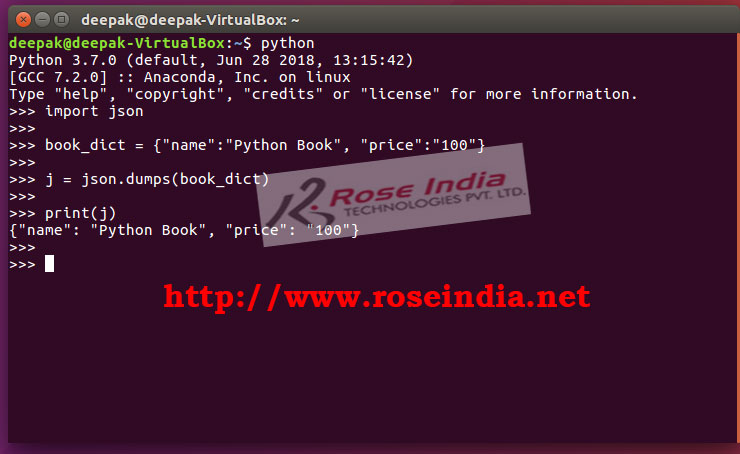Ads

Tutorials

# Convert dictionary to JSON Python

In this tutorial you will learn to Convert dictionary to JSON Python.

In this tutorial you will learn to Convert dictionary to JSON Python.

## Converting dictionary object to JSON in Python program

Dictionary is one of the most used data structure in Python. It allows you to store values in the form of key value pair. In dictionary key is unique and values are stored by key. In this tutorial we are going to see the python code for converting a dictionary object to JSON string.

These days JSON is used for developing applications for sending data over the internet and exchanging the data between applications. The JSON is preferred way to transfer data in applications. If you are working on web applications you can send data to your client browser in JSON format and then on the client side it can be easily manipulated using JavaScript library.

Consider the following dictionary:

``````
book_dict = {"name":"Python Book", "price":"100"}
```
```

In the above code we are declaring a dictionary in Python with two key and associated values. Now we want to convert it to JSON string. For converting dictionary to JSON string with the help of dumps() functions of json.

Here is the the complete code example:

``````
import json

book_dict = {"name":"Python Book", "price":"100"}

j = json.dumps(book_dict)

print(j)
```
```

If you run the above code it will print following data:

``````
{"name": "Python Book", "price": "100"}
```
```

Here is the screenshot of the example:In the above example we can see that the JSON output is not formatted. The json.dumps() function provides the way for formatted output. Here is example:

``````
import json

book_dict = {"name":"Python Book", "price":"100"}

j = json.dumps(book_dict,indent=4)

print(j)
```
```

This program will give following formatted output:

``````
>>> print(j)
{
"name": "Python Book",
"price": "100"
}
>>>
``````

The json.dumps() is used to convert dictionary object to JSON string in Python. In this example also we have used josn.dumps() function to convert it to JSON string.

If you want to sort the the data in created json by key then you can use following code:

``````
import json

book_dict = {"name":"Python Book", "price":"100", "availability":"available"}

j = json.dumps(book_dict,indent=4,sort_keys=True)

print(j)
``````

Here is output of the program:

``````
>>> print(j)
{
"availability": "available",
"name": "Python Book",
"price": "100"
}
>>>
``````

In this tutorial we have learned the code to convert dictionary to JSON string using various formatting options.

Ads

Ads